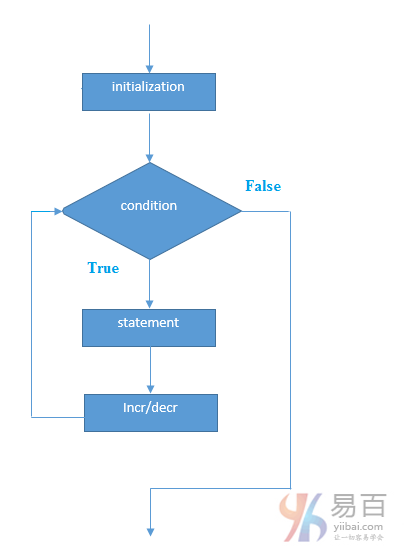# Java for循环

Java for循环用于多次迭代程序的一部分，或者多次执行同一个代码块。如果迭代次数是固定的，建议使用`for`循环。

## 更多精彩内容以及学习资料，尚学堂论坛bbs.bjsxt.com免费下载。

java中有三种类型的`for`循环。如下所示 -

• 简单`for`循环
• `for-each`或增强型`for`循环
• 标记`for`循环

## 1. Java简单For循环

` `for(initialization;condition;incr/decr){       //code to be executed   } ``
Java` `public class ForExample {       public static void main(String[] args) {           for(int i=1;i<=10;i++){               System.out.println(i);           }       }   } ``
Java

` `1 2 3 4 5 6 7 8 9 10 ``
Java

## 2. Java for-each循环

`for-each`循环用于在java中遍历数组或集合。它比简单的`for`循环更容易使用，因为不需要递增值和使用下标符号。

` `for(Type var:array){      //code to be executed   } ``
Java

` `public class ForEachExample  {     public static void main(String[] args) {         int arr[] = { 12, 23, 44, 56, 78 };         for (int i : arr) {             System.out.println(i);         }     } } ``
Java

` `12 23 44 56 78 ``
Java

## 3. Java标记For循环

` `labelname:   for(initialization;condition;incr/decr){       //code to be executed   } ``
Java

` `public class LabeledForExample  {     public static void main(String[] args) {         aa: for (int i = 1; i <= 3; i++) {             bb: for (int j = 1; j <= 3; j++) {                 if (i == 2 && j == 2) {                     break aa;                 }                 System.out.println(i + " " + j);             }         }     } } ``
Java

` `1 1 1 2 1 3 2 1 ``
Shell

` `public class LabeledForExample {     public static void main(String[] args) {         aa: for (int i = 1; i <= 3; i++) {             bb: for (int j = 1; j <= 3; j++) {                 if (i == 2 && j == 2) {                     break bb;                 }                 System.out.println(i + " " + j);             }         }     } } ``
Java

` `1 1 1 2 1 3 2 1 3 1 3 2 3 3 ``
Shell

## 4. Java无限循环

`for`循环中，如果使用两个分号`;`，则它对于循环将是不定式的。

` `for(;;){       //code to be executed   } ``
Java

` `public class ForExample {     public static void main(String[] args) {         for (;;) {             System.out.println("infinitive loop");         }     } } ``
Java

` `infinitive loop infinitive loop infinitive loop infinitive loop infinitive loop ctrl+c ``
Java

## 相关资讯

• #### 学堂故事

•尚学堂微博尚学堂订阅号尚学堂服务号

• 北京校区
• 西安校区
• 山西校区
• 武汉校区
• 深圳校区
• 上海校区
• 广州校区
• 保定招生办
• 黑龙江项目办

Copyright 2006-2021 北京尚学堂科技有限公司  京ICP备13018289号-19  京公网安备11010802015183   营业执照